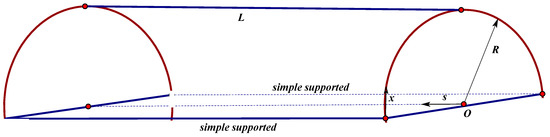Open AccessArticle

A New Analytical Prediction for Energy Responses of Hemi-Cylindrical Shells to Explosive Blast Load

1
School of Architecture and Civil Engineering, Jiangsu University of Science and Technology, Zhenjiang 212000, China
2
Department of Civil Engineering, The University of Birmingham, Birmingham B15 2TT, UK
*
Author to whom correspondence should be addressed.
Buildings 2019, 9(7), 168; https://doi.org/10.3390/buildings9070168
Received: 23 June 2019 / Revised: 4 July 2019 / Accepted: 5 July 2019 / Published: 11 July 2019
This study presents a new analytical model on the dissipation process of the initial total energy of the hemi-cylindrical shell subjected to the explosive blast load. The analytical formulation has been established using the energy method. The analytical predictions have been validated and found to be in excellent agreement with numerical simulations calculated by explicit finite element method (via LS-DYNA). The variational parameters considered are the shell thickness, elastic modulus, densities of the shell, and the positions of the detonation. Considering varieties of the parameters, the analytical and numerical results demonstrate that the pattern of vibrating deformations can be classified into two types according to the detonation positions. If the detonation position was at the midpoint of the width, there was no main frequency, whilst if the detonation position was at the edge of the width, the shell vibrated with a main frequency. It was also found from both analytical and numerical models that the total initial energy is inversely proportional to the thickness of the shell ( $T$ ), namely, the exact formula can be written as $β = ρ a c / ρ s T$ . Surprisingly, this study is the first to highlight that the total energy decreases with time by the exponential function, and the exponential ratio ( $β$ ) is inversely proportional to the thickness of the shell as well. View Full-Text
Show FiguresFigure 1

MDPI and ACS Style

Liu, P.; Xu, N.; Pan, Z.-H. A New Analytical Prediction for Energy Responses of Hemi-Cylindrical Shells to Explosive Blast Load. Buildings 2019, 9, 168.

Show more citation formats Show less citations formats
Note that from the first issue of 2016, MDPI journals use article numbers instead of page numbers. See further details here.

1